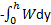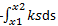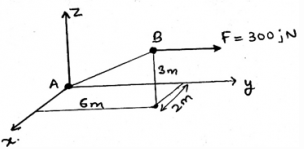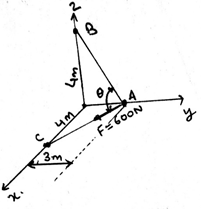Courses

# Test: Potential Energy

## 15 Questions MCQ Test Engineering Mechanics | Test: Potential Energy

Description
This mock test of Test: Potential Energy for Mechanical Engineering helps you for every Mechanical Engineering entrance exam. This contains 15 Multiple Choice Questions for Mechanical Engineering Test: Potential Energy (mcq) to study with solutions a complete question bank. The solved questions answers in this Test: Potential Energy quiz give you a good mix of easy questions and tough questions. Mechanical Engineering students definitely take this Test: Potential Energy exercise for a better result in the exam. You can find other Test: Potential Energy extra questions, long questions & short questions for Mechanical Engineering on EduRev as well by searching above.
QUESTION: 1

### Potential energy is stored in the body if some work is done on it. Work done is best given by:

Solution:

The work is defined as the dot product of the force and the distance. This means that the work done does depends upon the angle of the force. That is the angle which is being made by the force vector to the surface of action of the force.

QUESTION: 2

### If a block moves from a height h above the ground then the potential energy is given by_____

Solution:

The potential energy is converted into the work done. Thus is the block moves from the height h to the ground, the work done is given byNegative because the work done is in the opposite direction of the motion of the body.

QUESTION: 3

### Potential energy stored in a spring to a body from moving it from x1 to x2 distances is given by_____

Solution:

The potential energy is converted into the work done. Thus is the block moves from the distance x2 to x1, the work done is given byNegative because the work done is in the opposite direction of the motion of the body.

QUESTION: 4

For equilibrium the net moment acting on the body by various forces is zero, in any of the body in the space, as in the space it is acted by various forces, like gravity.

Solution:

The equilibrium is only attained if the net moment on the body tends to be equal to zero. Thus the moments caused by different forces cancels out. If this happens there is no motion of the body along any direction and hence the body is said to be in equilibrium. The body here is a rigid body.

QUESTION: 5

We use sometimes the measures to know the direction of moment in the calculations of the moments caused by the gravitational forces over the body above the ground level. Which one is right about it(consider the mentioned axis to be positive)?

Solution:

As right handed coordinate system means that you are curling your fingers from positive x-axis towards y-axis and the thumb which is projected is pointed to the positive z-axis. Thus visualizing the same and knowing the basic members of axis will not create much problem.

QUESTION: 6

Determine the magnitude of the force F = 300j parallel to the direction of AB?Solution:

Force component in the direction parallel to the AB is given by unit vector 0.286i + 0.857j + 0.429k. Now (300j).(0.286i + 0.857j + 0.429k) = 257.1N. Just try to resolve the force into it’s particular components.

QUESTION: 7

Which statement is correct about the vector F in the showing the gravitational nature of the forces?

Solution:

As we know the α, β and γ are the angles made by the x, y and z-axis respectively. Thus, is the magnitude of the vector is F, the F= Fcos β + Fcos α + Fcosγ. Which means the force is the resultant of all its axis’ components.

QUESTION: 8

What is the value of θ in the figure given below ?Solution:

Fab= -(3/5)j + (4/5)k ; F= (4/5)i – (3/5)j ; θ = cos-1(Fab.F) = 69˚. This is the application of the triangle over the figure. Try to resolve the components of the given force. It will be easy.

QUESTION: 9

Air also resist the body in the motion. The maximum value of the frictional force is called _________

Solution:

The friction is the phenomena that defines that there is a resistance which is present there between the two surfaces. The two surfaces are in contact and the friction applies at that surface only, resisting the motion of the surface. Thus the maximum values is called as limiting friction.

QUESTION: 10

The coefficient of kinetic friction when a body is moving from a height above the ground level to a smaller height is ____________ than coefficient of static friction.

Solution:

The coefficient of kinetic friction is smaller than coefficient of static friction. The main thing about the kinetic one is that it is applied by the surface when the body is in motion. The static one is applied to the body when the body is static and is about to move.

QUESTION: 11

Free body diagrams doesn’t play any role in making the calculations on the conditions of the equilibrium of the body when there is the involvement of the potential energy.

Solution:

The free body diagrams does play an important role in the formation of the conditions of the equilibrium of the rigid body. As the net forces are zero, the fbd helps us to take the measure of the same. That is to see whether the summation is really zero or not.

QUESTION: 12

The net forces of acting on the body needs to be zero. This is also applicable for the bodies having some potential energy. This means that the work done over it is saved in the form of potential energy.

Solution:

The support reactions of the beam is also counted in the making of the forces zero. As far as the net force is concerned the support reaction does affect the conditions for the equilibrium of the body. Hence one needs to take care of the support reactions of the beam too.

QUESTION: 13

Virtual Work done is saved in the form of potential energy. It is best given by ___________

Solution:

The work is defined as the dot product of the δ force and the δ distance. This means that the work done does depends upon the angle of the force. That is the angle which is being made by the force vector to the surface of action of the force.

QUESTION: 14

Which of the following is correct for the forces which are considered in the potential energy calculations?

Solution:

The application of the conditions of the equilibrium of the body is valid throughout. This means that the conditions are irrespective of the types of forces. The conditions are the basic rules that defines the equilibrium of the body and thus are applicable in any type of forces of the real axis.

QUESTION: 15

For the conditions of the equilibrium of the body, i.e. the rigid body only the external forces defines the equilibrium. And the support reactions only cancels out the rotation part of the body and thus the potential energy of the body is stored in it.

Solution:

The application of the support reaction forces does affect the conditions of the equilibrium of the body. Not only the external but the support reaction forces that are developed by the sake of external forces does develop a tending effect on the equilibrium of the body. Thus the support reaction forces also cancels the external forces.